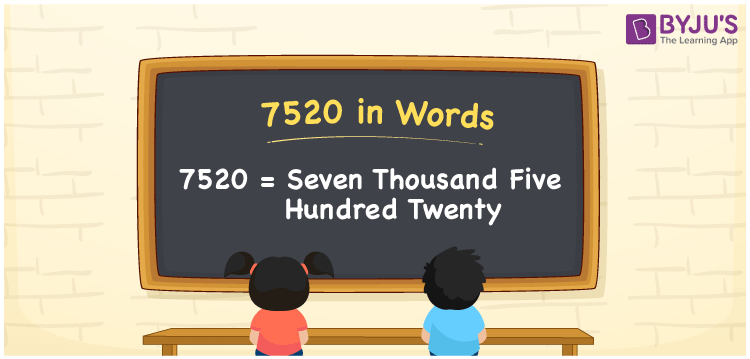# 7520 in words

7520 in words is written as Seven Thousand Five Hundred and Twenty. 7520 represents the count or value. The article on Counting Numbers can give you an idea about count or counting. The number 7520 is used in expressions that relate to money, distance, length and others. Let us consider an example for 7520. ”My first salary was Seven Thousand Five Hundred and Twenty rupees.”

 7520 in words Seven Thousand Five Hundred and Twenty Seven Thousand Five Hundred and Twenty in Numbers 7520

## 7520 in English Words## How to Write 7520 in Words?

We can convert 7520 to words using a place value chart. The number 7520 has 4 digits, so let’s make a chart that shows the place value up to 4 digits.

 Thousands Hundreds Tens Ones 7 5 2 0

Thus, we can write the expanded form as:

7 × Thousand + 5 × Hundred + 2 × Ten + 0 × One

= 7 × 1000 + 5 × 100 + 2 × 10 + 0 × 1

= 7520

= Seven Thousand Five Hundred and Twenty.

7520 is the natural number that is succeeded by 7519 and preceded by 7521.

7520 in words – Seven Thousand Five Hundred and Twenty.

Is 7520 an odd number? – No.

Is 7520 an even number? – Yes.

Is 7520 a perfect square number? – No.

Is 7520 a perfect cube number? – No.

Is 7520 a prime number? – No.

Is 7520 a composite number? – Yes.

## Solved Example

1. Write the number 7520 in expanded form

Solution: 7 × 1000 + 5 × 100 + 2 × 10 + 0 × 1

We can write 7520 = 7000 + 500 + 20 + 0

= 7 × 1000 + 5 × 100 + 2 × 10 + 0 × 1

## Frequently Asked Questions on 7520 in words

Q1

### How to write 7520 in words?

7520 in words is written as Seven Thousand Five Hundred and Twenty.
Q2

### Is 7520 a perfect square number?

No. 7520 is not a perfect square number.
Q3

### Is 7520 a prime number?

No. 7520 is not a prime number.